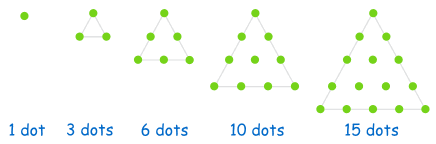# Python with Mathematics and Sequences – PART 1

Sequence is a particular order in which related things follow each other. Its fun and its very intuitive and so called logical. So lets start.

NOTE: Here we are not going to discuss what are different types of sequences are. We will see how we can achieve different sequences using Python.

Geometric Sequence

In a Geometric Sequence each term is found by multiplying the previous term by a constant.

2, 4, 8, 16, 32, 64, 128, 256, …

This sequence has a factor of 2 between each number. Each term (except the first term) is found by multiplying the previous term by 2.

```def gterm(n, cons):
x = 1
for i in range(1,n):
x = x * cons
print x
```

Arithmetic Sequences

In an Arithmetic Sequence the difference between one term and the next is a constant.

1, 4, 7, 10, 13, 16, 19, 22, 25, …

This sequence has a difference of 3 between each number.
Its Rule is xn = 3n-2

```>>> print range(1, 26, 3)
[1, 4, 7, 10, 13, 16, 19, 22, 25]
```

Triangular Numbers

The Triangular Number Sequence is generated from a pattern of dots which form a triangle.

1, 3, 6, 10, 15, 21, 28, 36, 45, …

By adding another row of dots and counting all the dots we can find the next number of the sequence:triangular numbers

But it is easier to use this Rule: xn = n(n+1)/2

```def triangle_numbers(n):
return [i*(i+1)/2 for i in xrange(n)]

>>> triangle_numbers(10)
[0, 1, 3, 6, 10, 15, 21, 28, 36, 45]
```

Square Numbers

The next number is made by squaring where it is in the pattern.

Rule is xn = n2

1, 4, 9, 16, 25, 36, 49, 64, 81, …

```def square_numbers(n):
return [i*i for i in xrange(1, 10)]

>>> square_numbers(10)
[1, 4, 9, 16, 25, 36, 49, 64, 81]
```

Cube Numbers

The next number is made by cubing where it is in the pattern.

Rule is xn = n3

1, 8, 27, 64, 125, 216, 343, 512, 729, …

```def cube_numbers(n):
return [i*i*i for i in xrange(1, 10)]

>>> cube_numbers(10)
[1, 8, 27, 64, 125, 216, 343, 512, 729]
```

Fibonacci Sequence

In this sequence the next number is found by adding the two numbers before it together.

Rule is xn = xn-1 + xn-2

0, 1, 1, 2, 3, 5, 8, 13, 21, 34, …

```def F(n):
if n == 0: return 0
elif n == 1: return 1
else: return F(n-1)+F(n-2)
```

Palindrome

A palindrome is a word, phrase, number, or other sequence of symbols or elements that reads the same forward or reversed, with general allowances for adjustments to punctuation and word dividers. Famous examples include “Amor, Roma”, “A man, a plan, a canal: Panama”, “Race car”, “Taco cat” and “No ‘x’ in ‘Nixon'”.

```>>> name = "Abhilash"
>>> name[::-1]
'hsalihbA'
```

Anagram

An anagram is a type of word play, the result of rearranging the letters of a word or phrase to produce a new word or phrase, using all the original letters exactly once; for example Doctor Who can be rearranged into Torchwood.

```def isAnagram(str1, str2):
str1_list = list(str1)
str1_list.sort()
str2_list = list(str2)
str2_list.sort()

return (str1_list == str2_list)
```

Pangram

Pangram is a sentence using every letter of the alphabet at least once. The best known English pangram is:

“The quick brown fox jumps over the lazy dog.”

```import string, sys
if sys.version_info < 3:
input = raw_input

def ispangram(sentence, alphabet=string.ascii_lowercase):
alphaset = set(alphabet)
return alphaset <= set(sentence.lower())

print ( ispangram(input('Sentence: ')) )
```

Prime Numbers

A prime number (or a prime) is a natural number greater than 1 that has no positive divisors other than 1 and itself.

2, 3, 5, 7, 11, 13, 17, 19, 23, 29, 31, 37, 41, 43, 47, …

```import math
for num in range(3,101,2):
if all(num%i!=0 for i in range(2,int(math.sqrt(num))+1)):
print num
```

Matrix

In mathematics, a matrix (plural matrices) is a rectangular array of numbers, symbols, or expressions, arranged in rows and columns.Each element of a matrix is often denoted by a variable with two subscripts. For instance, a2,1 represents the element at the second row and first column of a matrix A.
```>>> import numpy as np
>>> Y = np.array(list("ABCDE"*5)).reshape(5,5)
>>> print Y
[['A' 'B' 'C' 'D' 'E']
['A' 'B' 'C' 'D' 'E']
['A' 'B' 'C' 'D' 'E']
['A' 'B' 'C' 'D' 'E']
['A' 'B' 'C' 'D' 'E']]
>>> print Y.transpose()
[['A' 'A' 'A' 'A' 'A']
['B' 'B' 'B' 'B' 'B']
['C' 'C' 'C' 'C' 'C']
['D' 'D' 'D' 'D' 'D']
['E' 'E' 'E' 'E' 'E']]

# Another way
>>> matrix = [['A', 'B', 'C', 'D', 'E'], ['A', 'B', 'C', 'D', 'E'], ['A', 'B', 'C', 'D', 'E'], ['A', 'B', 'C', 'D', 'E'], ['A', 'B', 'C', 'D', 'E']]
>>> print 'n'.join([' '.join(row) for row in matrix])
```

That’s fun, right. Keep checking I will post the Part – 2 as soon as possible. Also, give me your feedback on Twitter or post me a DM from here.